Published: 01 December 2017

# Dynamic displacement estimation using data fusion

Sabine Upnere1
Normunds Jekabsons2
1Technical University, Institute of Mechanics, Riga, Latvia
1Ventspils University College, Ventspils, Latvia
2University of Latvia, Faculty of Physics and Mathematics, Riga, Latvia
Corresponding Author:
Sabine Upnere
Views 320

#### Abstract

The paper describes a Kalman filtering technique for dynamic displacement estimation using accelerometer and laser sensor measurements. Data fusion of measurements from multiple sensors can give the more accurate results because of different advantages of sensors. Since the acceleration and displacement have different sampling rates, the multi-rate Kalman filter is applied. The filter is expanded with the fixed interval smoother to improve reconstruction accuracy of displacements. A modelled signal consisting of two sinus functions and Gaussian distributed noise is used to validate developed state-space model.

## 1. Introduction

The vibration analysis is useful for the surveillance of a structure safety and structural health monitoring. The high-velocity fluid flow interaction with structural components can induce self-excited vibrations of the system elements in many engineering applications, for example, water-cooling systems or heat exchangers. In the exploration process of flow-induced vibrations in the rod bundle, two main interesting parameters are the rod oscillation frequency depending on surrounding flow velocity and the relationship between the rod position and the flow velocity.

Most commonly measured parameter for the characterisation of the structure response on the flow excitation is acceleration due to its relatively simple implementation. Using direct numerical double-integration of acceleration measurements to obtain displacements have to encounter with an integration error, which is not linearly accumulated during integration . Traditional methods, such as baseline correction techniques, two-baseline schemes or others, usually cannot give acceptable accuracy to evaluate displacements in the case when the mean value of acceleration measurements is non-zero or there are nonlinearities in displacements .

An alternative approach is to apply techniques of Kalman filters  analysing data from multiple sensors. In particular case, accelerometers and laser sensors are used to describe dynamics of a structure. Data fusion of measurements from different sensors can give the more accurate results, for example, accelerometer provides a better description of high-frequency process whereas laser sensors are more suitable to explore the low-frequency range. Due to sensors specific, the measurements of laser sensor and accelerometer are usually taken at different sampling rates. Smyth and Wu  used multi-rate Kalman filter data fusion to evaluate displacements. Measurement process was modelled using the state-space equation for velocity and acceleration. The technique of real-time estimation of dynamic displacement based on measured velocity and displacement from two different sensors are described by Kim and Sohn . They construct a state-space model for displacement and the total error.

In this paper is described multi-rate Kalman filter with fixed interval smoother for a state-space model for displacement, velocity and the accelerometer error. A modelled signal consisting of two sinus functions and Gaussian distributed noise is used to validate developed algorithm.

## 2. Displacement estimation using acceleration and displacement measurements

Non-contact accelerometer and laser sensors measurements can be done to analyse the flow velocity influence on the rod oscillation frequency and amplitude. Usually, accelerometer sensors have a higher sampling rate. However double integration leads to drift of the calculated mean value. The Kalman filter can be used to avoid an integration error. We assume that Kalman filter can be applied to each axis in the plane of the motion separately.

### 2.1. Double integration

It is assumed that the following mathematical model Eq. (1-2) can describe the rod movement in the discrete-time domain:

1
$\stackrel{˙}{x}\left(k+1\right)=\stackrel{˙}{x}\left(k\right)+\stackrel{¨}{x}\left(k\right)∆t+\epsilon \stackrel{¨}{x}\left(k\right)∆t,$
2
$x\left(k+1\right)=x\left(k\right)+\stackrel{˙}{x}\left(k\right)∆t+\frac{1}{2}\stackrel{¨}{x}\left(k\right){∆t}^{2}+\frac{1}{2}\epsilon \stackrel{¨}{x}\left(k\right){∆t}^{2},$

where $\stackrel{˙}{x}\left(k\right)$ and $x\left(k\right)$ are calculated velocity and displacement at the time step $k$, $∆t$ is the time interval for acceleration measurements and $\epsilon \stackrel{¨}{x}\left(k\right)$ is acceleration measurement error which is a combination of offset bias and zero-mean stochastic noise process, $w\left(k\right)$ .

### 2.2. Formulation of the Kalman filter

The dynamic displacement evaluates using displacement $x\left(k\right)$, velocity $\stackrel{˙}{x}\left(k\right)$ and error $\epsilon \stackrel{¨}{x}\left(k\right)$ as state variables:

3
$\mathbf{x}\left(k\right)=\left[\begin{array}{c}x\left(k\right)\\ \stackrel{˙}{x}\left(k\right)\\ \epsilon \stackrel{¨}{x}\left(k\right)\end{array}\right],$

Equations of the state-space model for multi-rate data fusion of laser and accelerometer measurements are as follows:

4
$\mathbf{x}\left(k\right)=\mathbf{A}\mathbf{x}\left(k-1\right)+\mathbf{B}\left(u\left(k-1\right)+w\left(k-1\right)\right),$
5
$\mathbf{z}\left(k\right)=\mathbf{H}\mathbf{x}\left(k\right)+v\left(k\right),$

where $u\left(k\right)$ is measured acceleration, $z\left(k\right)$ is measured displacement, $v\left(k\right)$ is measurement noise of a laser sensor and:

6
$\mathbf{A}=\left[\begin{array}{ccc}1& ∆t& 0.5{∆t}^{2}\\ 0& 1& ∆t\\ 0& 0& 1\end{array}\right],\mathbf{B}=\left[\begin{array}{c}0.5{∆t}^{2}\\ ∆t\\ 0\end{array}\right],\mathbf{H}=\left[\begin{array}{ccc}1& 0& 0\end{array}\right].$

The system equation Eq. (4) and observation equation Eq. (5) estimates in two steps: predictor, Eq. (7-8) and corrector Eq. (10-12):

7
${\mathbf{x}}_{fk}^{-}=\mathbf{A}{\mathbf{x}}_{f\mathbf{k}-1}^{+}+\mathbf{B}{\mathbf{u}}_{k-1},$
8
${\mathbf{P}}_{fk}^{-}=\mathbf{A}{\mathbf{P}}_{f\mathbf{k}-1}^{+}{\mathbf{A}}^{T}+\mathbf{Q},k=1,2,\dots ,N,$

where:

9
$\mathbf{Q}=q\mathbf{B}{\mathbf{B}}^{T},$

and $q$ is the covariance of the noise $w\left(k\right)$:

10
${\mathbf{x}}_{fk}^{+}={\mathbf{x}}_{fk}^{-}+{\mathbf{K}}_{fk}\left({\mathbit{z}}_{k}-{\mathbf{H}}_{k}{\mathbf{x}}_{fk}^{-}\right),$
11
${\mathbf{P}}_{fk}^{+}=\left(\mathbf{I}-{\mathbf{K}}_{fk}{\mathbf{H}}_{\mathbf{k}}\right){\mathbf{P}}_{fk}^{-},$
12
${\mathbf{K}}_{fk}={\mathbf{P}}_{fk}^{-}{\mathbf{H}}_{k}^{T}{\left({\mathbf{H}}_{k}{\mathbf{P}}_{fk}^{-}{\mathbf{H}}_{k}^{T}+r\right)}^{-1},$

where $r$ is the covariance of the measurement noise $v\left(k\right)$.

Since the acceleration and displacement have different sampling rates, the optimal estimates of the state variables can process by multi-rate Kalman filter . In time steps when displacement measurements are not available, only the prediction step is performed. The large sampling interval of the laser sensor can lead to drift of the displacement estimation. Smoothing can produce a better assessment .

Fixed-interval smoothing by Rauch-Tung-Striebel (RTS)  is used to correct the filtered signal. The RTS algorithm combines forward Kalman filtering and backward filtering Eqs. (15-17) with the following initialisation:

13
${\mathbf{x}}_{N}^{s}={\mathbf{x}}_{fk}^{+},{\mathbf{P}}_{N}^{s}={\mathbf{P}}_{fk}^{+},$
14
${\mathbf{x}}_{k}^{s}={\mathbf{x}}_{fk}^{+}+{\mathbf{K}}_{k}^{s}\left({\mathbf{x}}_{k+1}^{\mathbf{s}}-{\mathbf{x}}_{fk+1}^{-}\right),$
15
${\mathbf{P}}_{k}^{s}={\mathbf{P}}_{fk}^{+}-{\mathbf{K}}_{k}^{s}\left({\mathbf{P}}_{fk+1}^{-}-{\mathbf{P}}_{k+1}^{\mathbf{s}}\right){\left({\mathbf{K}}_{k}^{s}\right)}^{T},k=N-1,\dots ,0,$

where the smoother gain is:

16
${\mathbf{K}}_{k}^{s}={\mathbf{P}}_{fk}^{+}{\mathbf{A}}_{k}^{T}{\left({\mathbf{P}}_{fk+1}^{-}\right)}^{-1}.$

## 3. Test cases

Two modelled input signals one for displacements and one for acceleration are used to validate described algorithm. Dynamic displacement due to the flow-induced vibrations of the rod is estimated using data fusion of experimentally obtained acceleration and displacement measurements.

### 3.1. Case with modelled signal

For displacement simulation combination of two sinusoidal signals is used. The input of displacements is approximated as a quantized signal to resemble real measurements:

17
$x=-\frac{1}{{6\pi }^{2}}\mathrm{s}\mathrm{i}\mathrm{n}\left(6\pi t\right)-\frac{70}{{\left(80\pi \right)}^{2}}\mathrm{s}\mathrm{i}\mathrm{n}\left(80\pi t\right).$

Accelerometer signals obtained using the second-order derivative of the displacement Eq. (8). Noise with a normal distribution is applied to both input signals. The modelled signals can see in Fig. 1.

Fig. 1Modelled input signals: a) the laser sensor, b) accelerometer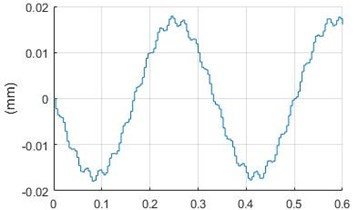a)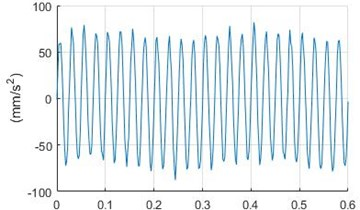b)

Fig. 2Calculated output signals: a) and corresponding error b)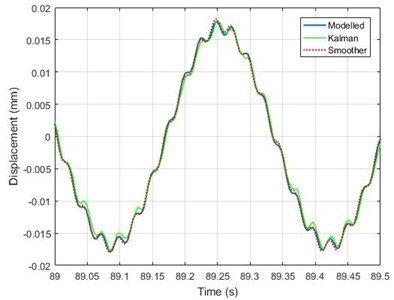a)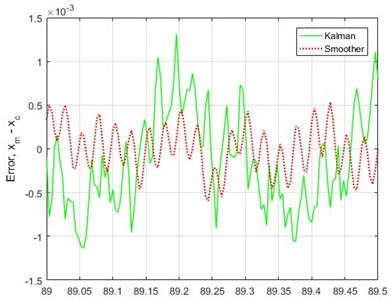b)

Displacement sampling time is two times larger than acceleration sampling. Example of filtered signals and the difference between modelled ${x}_{m}$ and filtered ${x}_{c}$ signals are shown in Fig. 2.

Maximum amplitudes of displacement using the filter without and with smoother at two noise levels are summarised in Table 1.

In all cases, the filter gives larger maximum amplitude as a modelled signal. Comparison of maximum values shows that reconstructions of the filter with smoother provide better agreement with expected values. Smoother decreases error approximately twice.

Table 1Comparison of maximum displacements depending on noise

 Maximum noise (% of maximum amplitude) Kalman filter(% of maximum amplitude) Kalman filter with smoother(% of maximum amplitude) 13.09 +4.6 +2.0 13.66 +3.1 +1.5 13.75 +4.4 +2.0 26.04 +6.9 +3.4 24.86 +8.6 +5.1 27.83 +6.5 +4.1

### 3.2. Experimental case

Experimental session was started with measurements at zero flow rate. Thus we presume that only noise was presented in the time signal. Typical distributions of the signal (noise) measured by accelerometers (Acc3 and Acc4) are shown in Fig. 3. In all presented cases the signal distribution is similar to Gaussian distribution (red line in the figure).

Fig. 3Signal (noise) distribution using experimental accelerometer data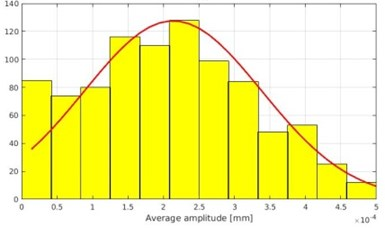a) Acc3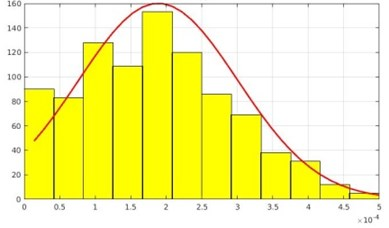b) Acc4

Displacements calculations using acceleration time history can also be done by so-called Omega Arithmetic (OA) method . There are found no differences between displacement reconstruction using multi-rate Kalman filter or OA when flow velocity is steady. However, multi-rate Kalman filter, unlike OA, can reconstruct the rod displacements in the unsteady regime when flow velocity is slowly increasing or decreasing in time, see Fig. 4.

Fig. 4Reconstruction of displacement: a) when flow velocity is increasing using multi-rate Kalman filter, b) omega arithmetic algorithm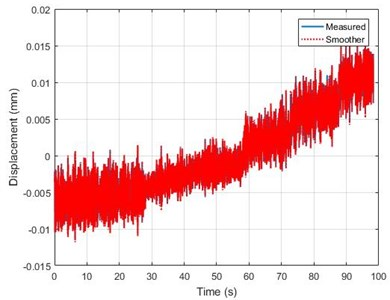a)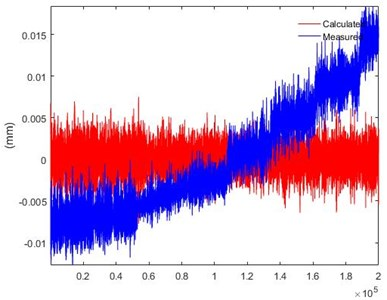b)

## 4. Conclusions

The fusion of an acceleration and displacement measurements using smoothing based multi-rate Kalman filter to estimate dynamic displacement is implemented and tested. Validation of proposed method is done by a modelled signal with additional normal distributed noise. Comparison of obtained results shows that smoother reduces reconstruction error approximately twice in given cases. The multi-rate Kalman filter can reconstruct time history when the flow velocity is slowly increased or decreased in unsteady flow rate measurements. The Omega Arithmetic method is less successful to reconstruct the rod coordinate oscillations in flow transients.

#### Cited by

Twin Rotor Damper for Human-Induced Vibrations of Footbridges

06 November 2017
Accepted
16 November 2017
Published
01 December 2017
SUBJECTS
Mathematical models in engineering
Keywords
Kalman filter
smoother
accelerometer sensor
laser sensors
sensor data fusion
Acknowledgements

Authors would like to express gratitude for experimental data to Dr. Sergejs Dementjevs from Paul Scherrer Institute.